Courses

Matrices part 1 Notes | Study Algebra for IIT JAM Mathematics - Mathematics

Mathematics: Matrices part 1 Notes | Study Algebra for IIT JAM Mathematics - Mathematics

The document Matrices part 1 Notes | Study Algebra for IIT JAM Mathematics - Mathematics is a part of the Mathematics Course Algebra for IIT JAM Mathematics.
All you need of Mathematics at this link: Mathematics

What is a matrix? Ever wondered what do the elements in a matrix mean? Why is it represented in a particular form? Why do we study a matrix? Does it really have any real-life application? I would say yes, matrices are the most important and are a basic part of maths which are used in higher studies and real-life problems.

Matrices are one of the most powerful tools in mathematics. The evolution of the concept of matrices is the result of an attempt to obtain compact and simple methods of solving the system of linear equations. Let’s find what is a matrix and its applications.

Definition of a Matrix
Matrix is an ordered rectangular arrangement of numbers (real or complex) or functions which may be represented as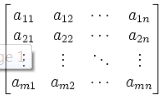Matrix is enclosed by [ ] or ( )

What is a Matrix?
Suppose we wish to express the information that Ram has 20 pens. We may express it as  with the understanding that the number inside [ ] is the number of pens that Ram has. Now, if we have to express that Ram has 20 pens and 7  pencils. We may express it as [20 7] with the understanding that first number inside [ ] is the number of pens while the other one is the number of pencils.

Let us now suppose that we wish to express the information of possession of pens and pencils by Ram and his two friends Rohan and Yash which is as follows:Now, this could be arranged in tabular form as follows,and this can be expressed as,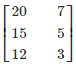In the above arrangement, the entries in the first column represent the number of pens possessed by Ram, Rohan, and Yash, respectively and the entries in the second column represents the number of pencils possessed by Ram, Rohan, and Yash, respectively.

Order of a Matrix
A matrix having m rows and n columns is called a matrix of order m × n or simply m × n matrix (read as an m by n matrix). In general, an m × n matrix has the following rectangular array;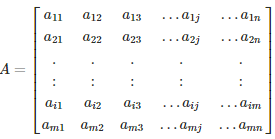Application of Matrices
Most Scientific Fields have Applications of Matrices in some or the other form. Almost every branch of physics, including classical mechanics, optics, electromagnetism, quantum mechanics, and quantum electrodynamics, matrices are used to study physical phenomena, such as the motion of rigid bodies.

In computer graphics, they are used to project a 3-dimensional image onto a 2-dimensional screen. In probability theory and statistics, stochastic matrices are used to describe sets of probabilities; for instance, they are used within the Page Rank algorithm that ranks the pages in a Google search.

Matrix calculus generalizes classical analytical notions such as derivatives and exponentials to higher dimensions. The graphics software uses the concept of a matrix to process linear transformations to render images.

Solved Examples For You
Question: If A = [1  2  3], then order is

1. 3 × 2
2. 3 × 1
3. 2 × 2
4. 1 × 3

Solution: An m×n matrix has m row and n columns. The given matrix A = [1  2  3] has 1 row and 3 columns. Thus, the order of A is 1 × 3. Hence, option D is correct.
Question: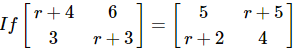• 1
• 2
• 3
• -1

Solution: We know that two matrices are equal iff their corresponding elements are equal. Thus comparing corresponding elements we get, for the first entry of the given matrices r + 4 = 5. Therefore r = 1. Hence, option A is correct.

Types of Matrices
Matrices are distinguished on the basis of their order, elements and certain other conditions. There are different types of matrices but the most commonly used are discussed below. Let’s find out the types of matrices in the field of mathematics.

Different types of Matrices and their forms are used for solving numerous problems. Some of them are as follows: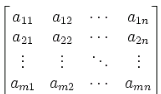1) Row Matrix
A row matrix has only one row but any number of columns. A matrix is said to be a row matrix if it has only one row. For example,
A=[−1/2 √5 2 3]
is a row matrix of order 1 × 4. In general, A = [aij]1 × n is a row matrix of order 1 × n.

2) Column Matrix
A column matrix has only one column but any number of rows. A matrix is said to be a column matrix if it has only one column. For example,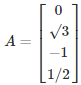is a column matrix of order 4 × 1. In general, B = [bij]m × 1 is a column matrix of order m × 1.

3) Square Matrix
A square matrix has the number of columns equal to the number of rows. A matrix in which the number of rows is equal to the number of columns is said to be a square matrix. Thus an m × n matrix is said to be a square matrix if m = n and is known as a square matrix of order ‘n’. For example,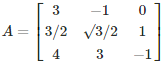is a square matrix of order 3. In general, A = [aij]m × m is a square matrix of order m.

4) Rectangular Matrix
A matrix is said to be a rectangular matrix if the number of rows is not equal to the number of columns. For example,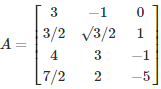is a matrix of the order 4 × 3

5) Diagonal matrix
A square matrix B = [bij] m × m is said to be a diagonal matrix if all its non-diagonal elements are zero, that is a matrix B =[bij]m×m is said to be a diagonal matrix if bij = 0, when i ≠ j. For example,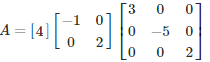are diagonal matrices of order 1, 2, 3, respectively.

6) Scalar Matrix
A diagonal matrix is said to be a scalar matrix if all the elements in its principal diagonal are equal to some non-zero constant. A diagonal matrix is said to be a scalar matrix if its diagonal elements are equal, that is, a square matrix B = [bij]n × n is said to be a scalar matrix if

• bij = 0, when i ≠ j
• bij = k, when i = j, for some constant k.

For example,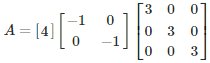are scalar matrices of order 1, 2 and 3, respectively.

7) Zero or Null Matrix
A matrix is said to be zero matrix or null matrix if all its elements are zero.
For Example,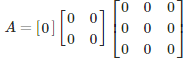are all zero matrices of the order 1, 2 and 3 respectively. We denote zero matrix by O.

8) Unit or Identity Matrix
If a square matrix has all elements 0 and each diagonal elements are non-zero, it is called identity matrix and denoted by I.

Equal Matrices: Two matrices are said to be equal if they are of the same order and if their corresponding elements are equal to the square matrix A = [aij]n × n is an identity matrix if

• aij = 1 if i = j
• aij = 0 if i ≠ j

We denote the identity matrix of order n by In. When the order is clear from the context, we simply write it as I. For example,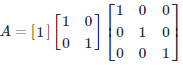are identity matrices of order 1, 2 and 3, respectively. Observe that a scalar matrix is an identity matrix when k = 1. But every identity matrix is clearly a scalar matrix.

9) Upper Triangular Matrix
A square matrix in which all the elements below the diagonal are zero is known as the upper triangular matrix. For example,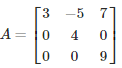10) Lower Triangular Matrix
A square matrix in which all the elements above the diagonal are zero is known as the upper triangular matrix. For example,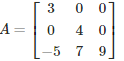Solved Examples For You
Question: Assertion :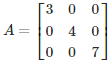is a diagonal matrix.

Reason: If A=[aij] is a square matrix such that aij=0,∀i≠j, then A is called a diagonal matrix.

1. Both Assertion and Reason are correct and Reason is the correct explanation for Assertion
2. Both Assertion and Reason are correct but Reason is not the correct explanation for Assertion
3. Assertion is correct but Reason is incorrect
4. Both Assertion and Reason are incorrect

Solution: If A=[aij]n×n is a square matrix such that aij = 0 for i≠j, then A is called a diagonal matrix. Since, a12 = a13 = a21 = a23 = a31 = a32 = 0 Thus, the given statement  is true and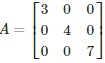is a diagonal matrix is a diagonal matrix

The document Matrices part 1 Notes | Study Algebra for IIT JAM Mathematics - Mathematics is a part of the Mathematics Course Algebra for IIT JAM Mathematics.
All you need of Mathematics at this link: MathematicsUse Code STAYHOME200 and get INR 200 additional OFF Use Coupon CodeAlgebra for IIT JAM Mathematics

70 videos|58 docs

Track your progress, build streaks, highlight & save important lessons and more!

,

,

,

,

,

,

,

,

,

,

,

,

,

,

,

,

,

,

,

,

,

;